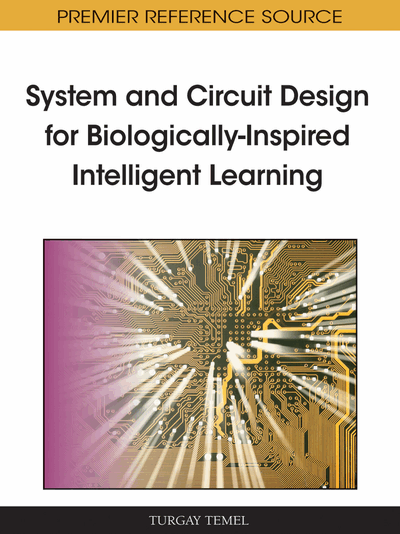# Parallel Hardware for Artificial Neural Networks Using Fixed Floating Point Representation

Nadia Nedjah (State University of Rio de Janeiro, Brazil), Rodrigo Martins da Silva (State University of Rio de Janeiro, Brazil) and Luiza de Macedo Mourelle (State University of Rio de Janeiro, Brazil)
DOI: 10.4018/978-1-60960-018-1.ch013
Available
\$37.50
No Current Special Offers

## Abstract

Artificial Neural Networks (ANNs) is a well known bio-inspired model that simulates human brain capabilities such as learning and generalization. ANNs consist of a number of interconnected processing units, wherein each unit performs a weighted sum followed by the evaluation of a given activation function. The involved computation has a tremendous impact on the implementation efficiency. Existing hardware implementations of ANNs attempt to speed up the computational process. However, these implementations require a huge silicon area that makes it almost impossible to fit within the resources available on a state-of-the-art FPGAs. In this chapter, a hardware architecture for ANNs that takes advantage of the dedicated adder blocks, commonly called MACs, to compute both the weighted sum and the activation function is devised. The proposed architecture requires a reduced silicon area considering the fact that the MACs come for free as these are FPGA’s built-in cores. Our system uses integer (fixed point) mathematics and operates with fractions to represent real numbers. Hence, floating point representation is not employed and any mathematical computation of the ANN hardware is based on combinational circuitry (performing only sums and multiplications). The hardware is fast because it is massively parallel. Besides, the proposed architecture can adjust itself on-the-fly to the user-defined configuration of the neural network, i.e., the number of layers and neurons per layer of the ANN can be settled with no extra hardware changes. This is a very nice characteristic in robot-like systems considering the possibility of the same hardware may be exploited in different tasks. The hardware also requires another system (a software) that controls the sequence of the hardware computation and provides inputs, weights and biases for the ANN in hardware. Thus, a co-design environment is necessary.
Chapter Preview
Top

## Introduction

Artificial Neural Networks (ANNs) are useful for learning, generalization, classification and forecasting problems (Hassoun, 1995). They consist of a pool of relatively simple processing units, usually called artificial neurons, which communicates with one another through a large set of weighted connections. There are two main network topologies, which are feed-forward topology (Hassoun, 1995; Moerland & Fiesler, 1996), where the data flows from input to output neurons strictly forward and recurrent topology, where feedback connections are allowed. Artificial neural networks offer an attractive model that allows one to solve hard problems from examples or patterns. However, the computational process behind this model is complex. It consists of massively parallel non-linear calculations. Software implementations of artificial neural networks are useful but hardware implementations take advantage of the inherent parallelism of ANNs and so should answer faster.

Field Programmable Gate Arrays (FPGAs) (Xilinx, 2009) provide a re-programmable hardware that allows one to implement ANNs very rapidly and at very low-cost. However, FPGAs lack the necessary circuit density as each artificial neuron of the network needs to perform a large number of multiplications and additions, which consume a lot of silicon area if implemented using standard digital techniques, as floating-point operations.

The proposed hardware architecture described throughout this chapter is designed to process any fully connected feed-forward Multilayer Perceptrons (MLP) neural network. However, training is not included. It is now a common knowledge that the computation performed by the net is complex and consequently has a huge impact on the implementation efficiency and practicality. Existing hardware implementations of ANNs have attempted to speed up the computational process. However these designs require a considerable silicon area that makes them almost impossible to fit within the resources available on a state-of-the-art FPGAs (Bade & Hutchings, 1994; Brown & Card, 2001; Nedjah & Mourelle, 2007; Seul; & Sung, 2007, Tuffy et. al., 2007; Harkin, et. al., 2009). In this chapter, an original hardware architecture for ANNs that takes advantage of the dedicated adder blocks, commonly called MACs (short for Multiply, Add and Accumulate blocks) to compute both the weighted sum and the activation function is proposed.

Our system uses a specific number representation: Fractional Fixed Point (Saint-Jones & Gu, 2003). It means that a real number is treated (approximated) by a fraction. Fractional addition, subtraction and multiplication are inherently integer (fixed point) operations, which may be an attractive choice in decreasing silicon area, because integer mathematics can be done by combinational circuitry.

The weighted sum of a neuron is now a sum of fractional products. In this project, the activation function (for all neurons of the ANN) is the sigmoidal logistic function (logsig), whose mathematics is also reduced (approximated) to additions, subtractions and multiplications of fractions. The exponential term exp(∙) of the logsig function is approximated to 3 quadratic polynomials, using least-squares parabola method.

The proposed architecture requires a reduced silicon area considering the fact that the MACs come for free as these are FPGA’s built-in cores. The hardware is fast because it is massively parallel. Besides, the proposed hardware can adjust itself on-the-fly to the user-defined configuration of the neural network, with no extra hardware changes, which is a very nice characteristic in robot-like systems considering the possibility of the same piece of hardware may be exploited in different tasks. This feature if the hardware is very used in bio-inspired learning application.

## Complete Chapter List

Search this Book:
Reset